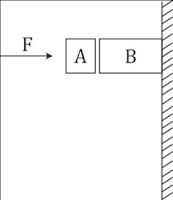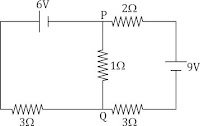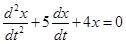#searchbox { width: 240px; } #searchbox input { outline: none; } input:focus::-webkit-input-placeholder { color: transparent; } input:focus:-moz-placeholder { color: transparent; } input:focus::-moz-placeholder { color: transparent; } #searchbox input[type="text"] { background: url(https://2.bp.blogspot.com/-vHUvznDs39w/Vp9e7ZqWC6I/AAAAAAAACSM/l2WCueYWFpE/s1600/search-dark.png) no-repeat 10px 13px #f2f2f2; border: 2px solid #f2f2f2; font: bold 12px Arial,Helvetica,Sans-serif; color: #6A6F75; width: 160px; padding: 14px 17px 12px 30px; -webkit-border-radius: 5px 0px 0px 5px; -moz-border-radius: 5px 0px 0px 5px; border-radius: 5px 0px 0px 5px; text-shadow: 0 2px 3px #fff; -webkit-transition: all 0.7s ease 0s; -moz-transition: all 0.7s ease 0s; -o-transition: all 0.7s ease 0s; transition: all 0.7s ease 0s; } #searchbox input[type="text"]:focus { background: #f7f7f7; border: 2px solid #f7f7f7; width: 200px; padding-left: 10px; } #button-submit{ background: url(https://2.bp.blogspot.com/-6z-Tib5VRS8/Vp9e7SahULI/AAAAAAAACSI/T-C3o550Jvo/s1600/slider-arrow-right.png) no-repeat; margin-left: -40px; border-width: 0px; width: 43px; height: 45px; cursor: pointer; text-indent: 100%; white-space: nowrap; overflow: hidden; }

### JEE Main

NOTE: Attempt all Questions to see the Result at the bottom of this page. The Timer has started 60:00

PHYSICS

1. 1)Given in the figure are two blocks A and B of weight 20 N and 100 N, respectively. These are being pressed against a wall by a force F as shown. If the coefficient of friction between the blocks is 0.1 and between block B and the wall is 0.15, the frictional force applied by the wall on block B is:

• A) 100 N
• B) 80 N
• C) 120 N
• D) 150 N

2. 2)

A particle of mass m moving in the x direction with speed 2v is hit by another particle of mass 2m moving in the y direction with speed v. If the collision is perfectly inelastic, the percentage loss in the energy during the collision is close to:

• A) 44 %
• B) 50 %
• C) 56 %
• D) 62 %

3. 3)

A red LED emits light at 0.1 watt uniformly around it. The amplitude of the electric field of the light at a distance of 1 m from the diode is:

• A) 1.73 V/m
• B) 2.45 V/m
• C) 5.48 V/m
• D) 7.75 V/m

4. 4)

A On a hot summer night, the refractive index of air is smallest near the ground and increases with height from the ground. When a light beam is directed horizontally, the Huygens' principle leads us to conclude that as it travels, the light beam:

• A) becomes narrower
• B) goes horizontally without any deflection
• C) bends downwards
• D) bends upwards

5. 5)

Assuming human pupil to have a radius of 0.25 cm and a comfortable viewing distance of 25 cm, the minimum separation between two objects that human eye can resolve at 500 nm wavelength is:

• A) 1 μm
• B) 30 μm
• C) 100 μm
• D) 300 μm

6. 6)

As an electron makes a transition from an excited state to the ground state of a hydrogen - like atom/ ion:

• A) its kinetic energy increases but potential energy and total energy decrease
• B) kinetic energy, potential energy and total energy decrease
• C) kinetic energy decreases, potential energy increases but total energy remains same
• D) kinetic energy and total energy decrease but potential energy increases

7. 7)

A signal of 5 kHz frequency is amplitude modulated on a carrier wave of frequency 2 MHz. the frequencies of the resultant signal is/are:

• A) 2 MHz only
• B) 2005 kHz, and 1995 kHz
• C) 2005 kHz, 2000 kHz and 1995 kHz
• D) 2000 kHz and 1995 kHz

8. 8)

Two coaxial solenoids of different radii carry current I in the same direction. Let  F1 be
the magnetic force on the inner solenoid due to the outer one and  F2 be the magnetic
force on the outer solenoid due to the inner one. Then:

• A) F1 = F2 = 0
• C) F1 is radially inwards and F2 = 0
• D) F1 is radially outwards and F2 = 0

9. 9)In the circuit shown, the current in the 1 Ω resistor is:

• A) 1.3 A from P to Q
• B) 0 A
• C) 0.13 A, from Q to P
• D) 0.13 A, from P to Q

10. 10)

A solid body of constant heat capacity 1 J/°C is being heated by keeping it in contact with reservoirs in two ways:
(i) Sequentially keeping in contact with 2 reservoirs such that each reservoir supplies same amount of heat.
(ii) Sequentially keeping in contact with 8 reservoirs such that each reservoir supplies same amount of heat.
In both the cases body is brought from initial temperature 100 °C to final temperature 200 °C. Entropy change of the body in the two cases respectively is:

• A) ln2, 4ln2
• B) ln2, ln2
• C) ln2, 2ln2
• D) 2ln2, 8ln2

11. CHEMISTRY

12. 11)

Sodium metal crystallizes in a body centred cubic lattice with a unit cell edge of 4.29 Å. The radius of sodium atom is approximately

• A) 1.86 Å
• B) 3.22 Å
• C) 5.72 Å
• D) 0.93 Å

13. 12)

Which of the following is the energy of a possible excited state of hydrogen?

• A) +13.6 eV
• B) –6.8 eV
• C) –3.4 eV
• D) –3.4 eV

14. 13)

The intermolecular interaction that is dependent on the inverse cube of distance between the molecules is

• A) Ion-ion interaction
• B) Ion-dipole interaction
• C) London force
• D) Hydrogen bond

15. 14)

The vapour pressure of acetone at 20°C is 185 torr. When 1.2 g of a non-volatile substance was dissolved in 100 g of acetone at 20°C, its vapour pressure was 183 torr. The molar mass of the substance is:

• A) 32
• B) 64
• C) 128
• D) 488

16. 15)

Two faraday of electricity is passed through a solution of CuSO4. The mass of copper deposited at the cathode is (at. mass of Cu = 63.5 amu)

• A) 0 g
• B) 63.5 g
• C) 2 g
• D) 127 g

17. 16)

Higher order (>3) reactions are rare due to

• A) Low probability of simultaneous collision of all the reacting species
• B) Increase in entropy and activation energy as more molecules are involved
• C) Shifting of equilibrium towards reactants due to elastic collisions
• D) Loss of active species on collision

18. 17)

3 g of activated charcoal was added to 50 mL of acetic acid solution (0.06N) in a flask. After an hour it was filtered and the strength of the filtrate was found to be 0.042 N. The amount of acetic acid adsorbed (per gram of charcoal) is

• A) 18 mg
• B) 36 mg
• C) 42 mg
• D) 54 mg

19. 18)

Which one of the following alkaline earth metal sulphates has its hydration enthalpy greater than its lattice enthalpy?

• A) CaSO4
• B) BeSO4
• C) BaSO4
• D) SrSO4

20. 19)

The color of KMnO4 is due to:

• A) M → L charge transfer transition
• B) d - d transition
• C) L → M charge transfer transition
• D) σ – σ* transition

21. 20)

50. Assertion: Nitrogen and Oxygen are the main components in the atmosphere but these do not react to form oxides of nitrogen.
Reason: The reaction between nitrogen and oxygen requires high temperature.

• A) Both assertion and reason are correct, and the reason is the correct explanation for the assertion
• B) Both assertion and reason are correct, but the reason is not the correct explanation for the assertion
• C) The assertion is incorrect, but the reason is correct
• D) Both the assertion and reason are incorrect

22. MATHEMATICS

23. 21)

Let A and B be two sets containing four and two elements respectively. Then the number of subsets of the set AB, each having at least three elements is:

• A) 219
• B) 256
• C) 275
• D) 510

24. 22)

The set of all values of λ for which the system of linear equations:
2x1- 2x2 + x3 = λx1
2x1 - 3x2 + 2x3 = λx2
-x1+2x2 = λx3
has a non-trivial solution,

• A) is an empty set.
• B) is a singleton.
• C) contains two elements.
• D) contains more than two elements.

25. 23)

The number of integers greater than 6,000 that can be formed, using the digits 3, 5, 6, 7 and 8, without repetition, is:

• A) 216
• B) 192
• C) 120
• D) 72

26. 24)

The number of points, having both co-ordinates as integers, that lie in the interior of the triangle with vertices (0, 0), (0, 41) and (41, 0), is:

• A) 901
• B) 861
• C) 820
• D) 780

27. 25)

Locus of the image of the point (2, 3) in the line (2x - 3y + 4) + k (x – 2y+3) = 0, k ε R, is a:

• A) straight line parallel to x-axis.
• B) straight line parallel to y-axis.
• C) circle of radius √2.
• D) circle of radius √3.

28. 26)

84. The equation of the plane containing the line 2x - 5y + z = 3, x + y + 4z = 5, and parallel to the plane, x + 3y + 6z = 1, is:

• A) 2x + 6y + 12z = 13
• B) x + 3y + 6z = -7
• C) x + 3y + 6z = 7
• D) 2x + 6y + 12z = -13

29. 27)

87. The mean of the data set comprising of 16 observations is 16. If one of the observation valued 16 is deleted and three new observations valued 3, 4 and 5 are added to the data, then the mean of the resultant data, is:

• A) 16.8
• B) 16.0
• C) 15.8
• D) 14.0

30. 28)

88. If the angles of elevation of the top of a tower from three collinear points A, B and C, on a line leading to the foot of the tower, are 30°, 45° and 60° respectively, then the ratio, AB : BC, is:

• A) √3:1
• B) √3: √2
• C) 1: √3
• D) 2:3

31. 29)

90. The negation of ~ S ⋁ (~ r ⋀ s) is equivalent to:

• A) s ⋀ ~ r
• B) s ⋀ (r ⋀ ~ s)
• C) s ⋁ (r ⋁ ~ s)
• D) s ⋀ r

32. 30)

The given differential equation• A) is Over damped
• B) is Critically damped
• C) is Under damped
• D) None of them